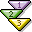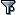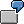Calling Up Calculation RowsYou can call up calculation rows for quantity determination or for activation.

1. From the function selection, choose the function CalculationRowResult (node: General Data).
2. Enter the name of the calculation row as a parameter (entry in the object column of the template table) that you want to call up.
3. Enter the type of the column in which you defined the formula (use the fixed values and F4 selection).
4. Define the remaining formula as usual.
1. In the editor, choose the function CalculationRowResult as comparison operand 1 (node: General Data).
2. Enter the name of the calculation row as a parameter (entry in the object column of the template table) that you want to call up.
3. Enter the type in the column in which you defined the formula (use the fixed values and F4 selection).
4. Select "=" or "Not equal" as the operator.
5. Choosefrom the function selection.
6. Select True or False as the functions for comparison operand 2.

The operator "=" activates the value of the item when the valuation event occurs if the values of the calculation row and comparison operand 2 are the same. The operator Not equal gives the opposite result.

Result of Method for Operator "="

 Comparison Operand 2 Result of Calculation Row Item Activation True True Active True False Inactive False True Inactive False False ActiveYou entered the function False for comparison operand 2. If the value of the calculation row at the time of the valuation event is also False, the result of the method is Active; If the calculation row returns the value True, the result of the method is Inactive.